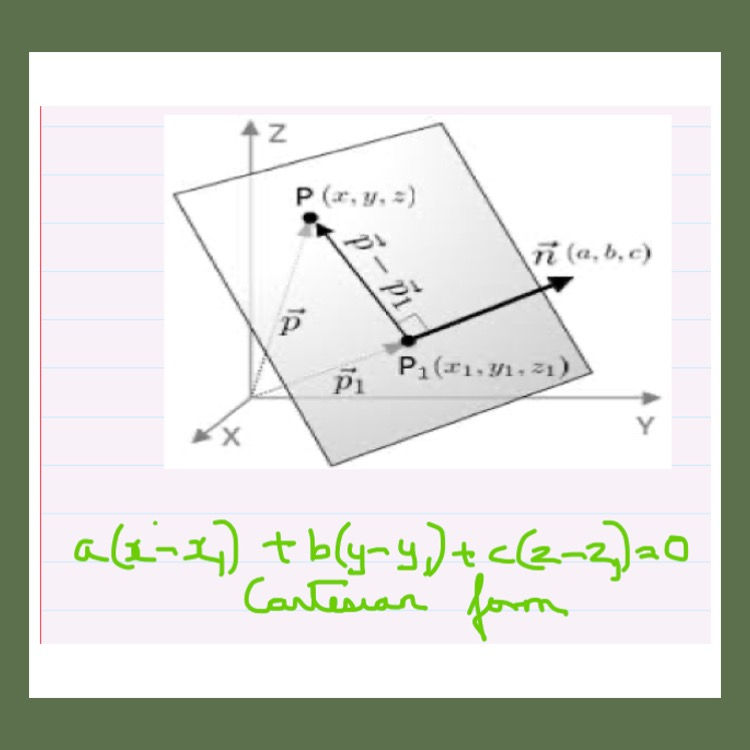top of page
Search

# Yes-The Quickest and Easiest Way For You to Understand Planes

## 3 D Geometry starts with the basic equation of a plane. You'll learn to write the basic equation of a plane given the unit normal and the length of a perpendicular from the origin to the plane. The following image helps illustrate this.Equation of a plane given the unit normal

## This formula is shown here and is extremely important for you when you are learning 3-D Geometry.Cartesian equation of a plane

## The following problem shows you how to find the direction cosines of the normal to the plane. You need to remember that in a plane ax+by+cz+d=0, <a,b,c> are the direction ratios of the normal to the plane.Direction cosines of the normal to the plane

## Finding the image of a point in a plane is yet another topic that is explained here. The following problem should throw some light on this.Image of a point in a plane